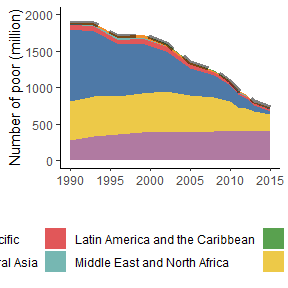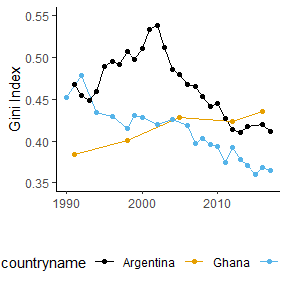# Visualization examples

library(povcalnetR)
library(ggplot2)
library(tidyr)
library(ggthemes)
library(forcats)
library(scales)
library(dplyr)

## Number of poor by region

df <- povcalnet_wb() %>%
filter(year > 1989) %>%
mutate(
poor_pop = round(headcount * population, 0),
)

regions <- df %>%
filter(regioncode != "WLD") %>%
mutate(
regiontitle = fct_relevel(regiontitle,
c("Other high Income",
"Europe and Central Asia",
"Middle East and North Africa",
"Latin America and the Caribbean",
"East Asia and Pacific",
"South Asia",
"Sub-Saharan Africa"
))
)
world <- df %>%
filter(regioncode == "WLD")

ggplot(regions, aes(y = poor_pop, x = year, fill = regiontitle)) +
geom_area() +
scale_y_continuous(
limits = c(0, 2000),
breaks = c(0, 500, 1000, 1500, 2000)
) +
scale_fill_tableau(palette = "Tableau 10") +
labs(
y = "Number of poor (million)",
x = ""
) +
theme_classic() +
theme(
legend.position = "bottom"
) +
geom_line(data = world, size = rel(1.5), alpha =.5, linetype = "longdash")## Gini Indices for Argentina, Ghana and Thailand

df <- povcalnet(country = c("ARG", "GHA", "THA"),
coverage = "all") %>%
filter(year > 1989) %>%
select(countrycode:isinterpolated, gini)

ggplot(df, aes(x = year, y = gini, color = countryname)) +
geom_line() +
geom_point(data = df[df\$isinterpolated == 0, ]) +
scale_y_continuous(
limits = c(0.35, 0.55),
breaks = c(0.35, 0.40, 0.45, 0.50, 0.55)
) +
scale_color_colorblind() +
labs(
y = "Gini Index",
x = ""
) +
theme_classic() +
theme(
legend.position = "bottom"
)